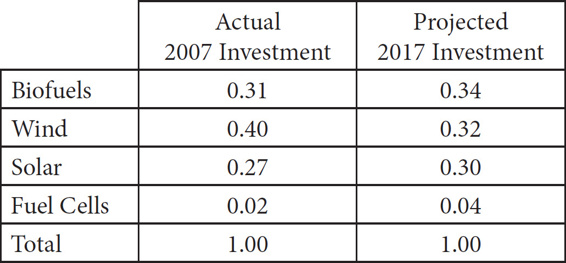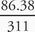# SAT Math Multiple Choice Question 97: Answer and Explanation

### Test Information

Question: 97

7. United States Investment in
Alternative Energy SourcesThe table above shows the relative investment in alternative energy sources in the United States by type. One column shows the relative investment in 2007 of \$75 million total invested in alternative energy. The other column shows the projected relative investment in 2017 given current trends. The total projected investment in alternative energy in 2017 is \$254 million. Suppose that a new source of alternative energy, Cold Fusion, is perfected. It is projected that by 2017 that \$57 million will be invested in Cold Fusion in the United States, without any corresponding reduction in investment for any other form of alternative energy. What portion of the total investment of alternative energy in the United States will be spent on biofuels?

• A. 0.18
• B. 0.22
• C. 0.28
• D. 0.34

C First, you know the new proportion must be less than the current 0.34 for biofuels (because the total amount spent on alternative energy is increasing, but the amount spent on biofuels is remaining the same), so you can eliminate (D). Next, determine the amount that will be spent on biofuels in 2017 by multiplying 0.34 by the total of \$254 million: 0.34 × 254 = \$86.36 million. Because 57 million new dollars will be spent on alternative energy, the new total will be 254 + 57 = \$311 million. Divide \$86.36 million by \$311 million to get the new proportion:= 0.28, which is (C).